﻿ Add, subtract, multiply, and divide in Excel

# Divide, subtract, add, and multiply in Excel

## Calculate percentage of a number

Apply the same math operations to all numbers in the selected range. Division, multiplication, subtraction, and addition are no longer a problem. Find percentage of a number, add percentages to your numbers, or decrease a number by percentage.

2019 2016 365 2013 2010 2007
This tool is part of
Ultimate Suite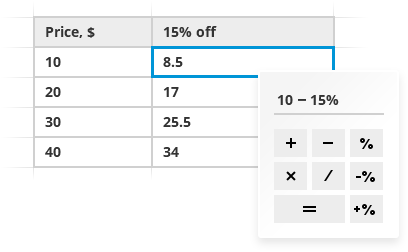## With Calculate Tool you will

 Calculate ranges in a click Apply the same math operation to all numbers in a range. Add and subtract Add or subtract a specific number from all selected cells. Calculate percentage in Excel Subtract or add percentage to each cell in a range or find the percentage of all numbers. Multiply and divide Multiply or divide individual cells and entire columns. Apply recent calculations Pick one of the last 10 operations to apply it again. Add new calculations to existing formulas Modify all current formulas in a range automatically based on the math operation you select.

### How to quickly calculate multiple cells in Excel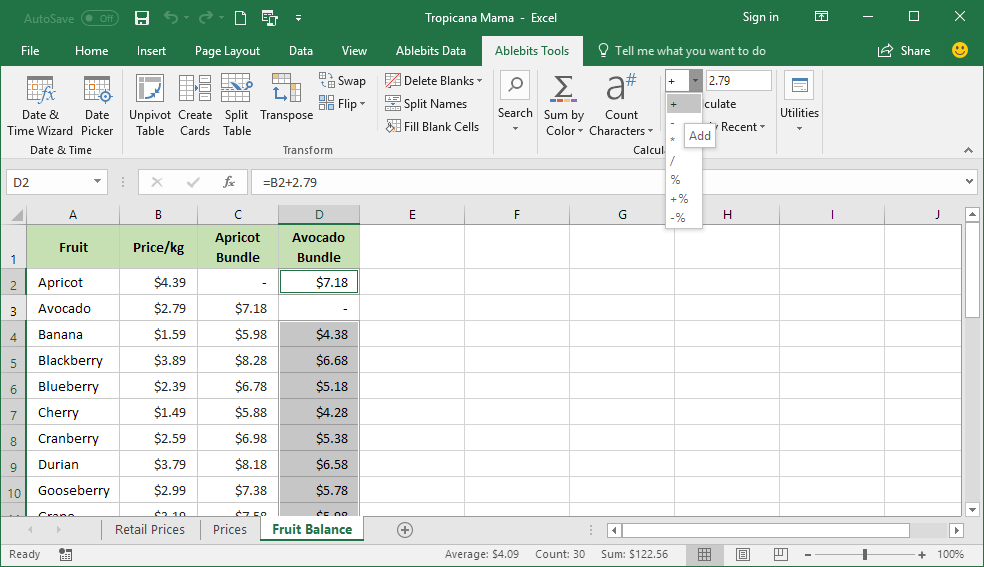How to add number to multiple Excel cells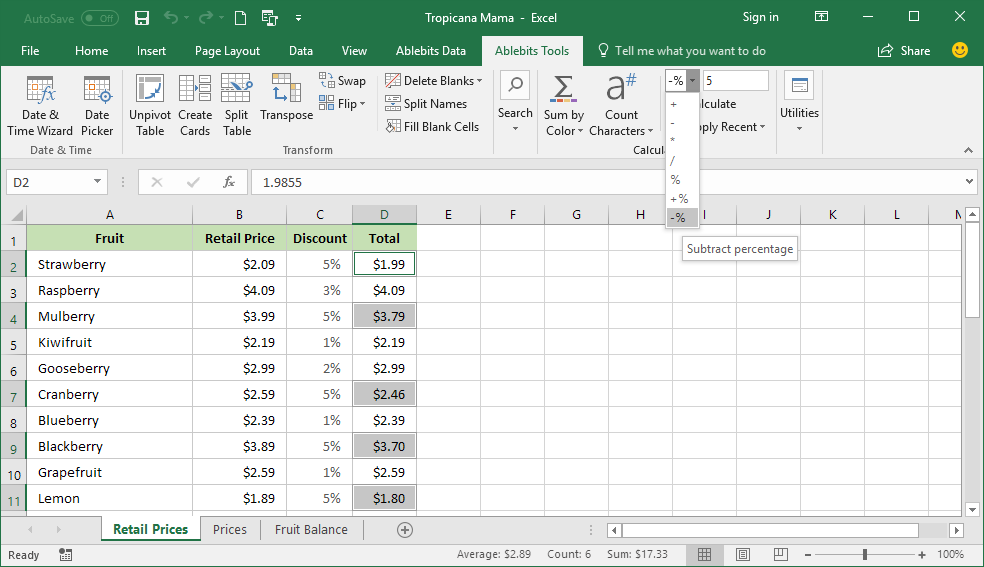How to subtract percentage from a number in Excel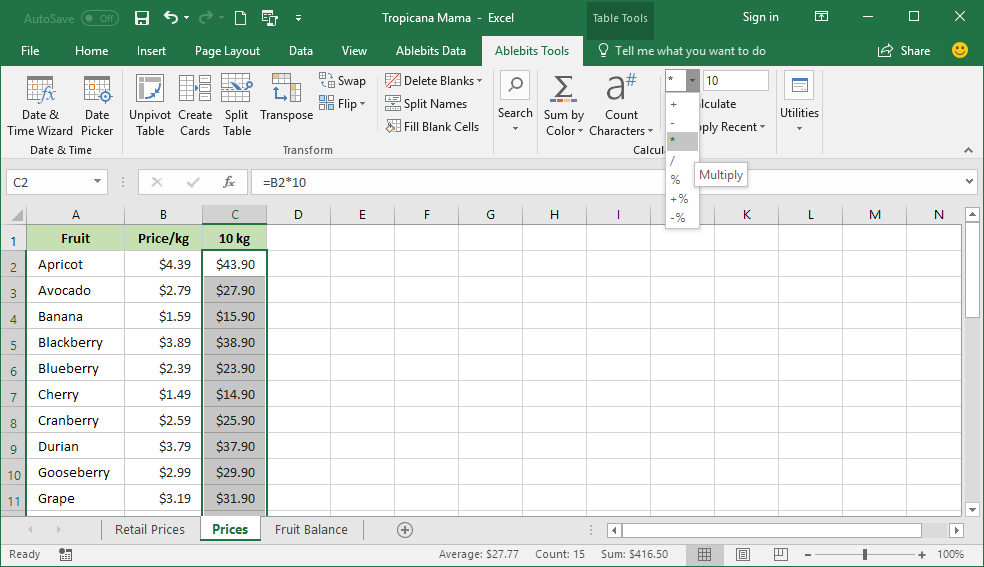Multiply all cells in a column at once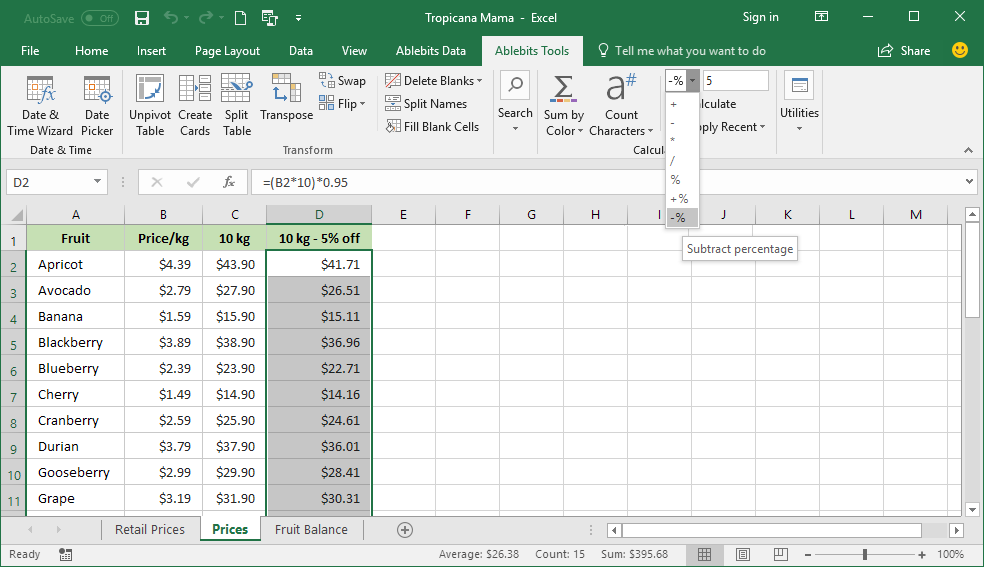Add one more operation to the existing formulas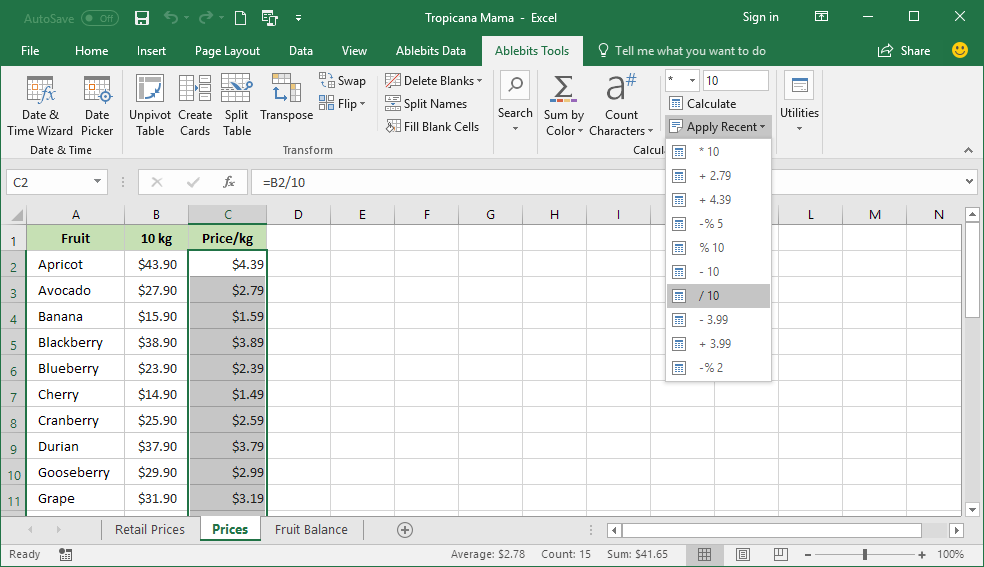Apply one of 10 last calculations again

### Our customers get

 Unconditional 60-day money-back guarantee Secure paymentguarantee Life-time license,no additional fees ever Volume discounts: the more you buy, the less you pay 1 license for 2 or 5 PCs or 1 server Free life-time support
 Learn more This tool is part of Ultimate Suite 60+ professional tools. No garbage. Buy now Buy as part of Ultimate Suite 60+ professional tools. No garbage.

## These companies choose Ablebits products

This tool is part of Ultimate Suite

## Supported versions

#### Excel

• Excel 2019
• Excel 2016
• Excel 2013
• Excel 2010
• Excel 2007
• 32-bit and 64-bit
• Desktop Excel included in Office 365
• Desktop Excel included in Office 365 ProPlus

#### Windows

• Windows 10
• Windows 8.1
• Windows 8
• Windows 7
• 32-bit and 64-bit
• Buy as part of Ultimate Suite
• 60+ professional tools. No garbage.